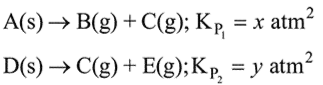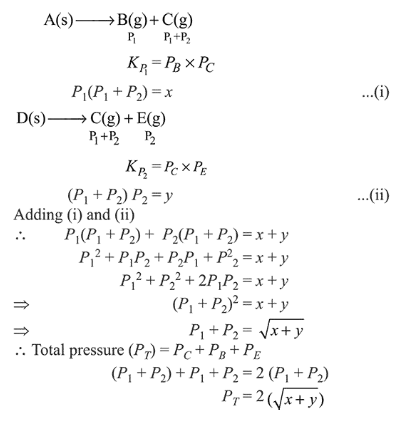# Two solids dissociate as followsQuestion:

Two solids dissociate as followsThe total pressure when both the solids dissociate simultaneously is:

1. $\sqrt{x+y}$ atm

2. $2(\sqrt{x+y})$ atm

3. $(x+y)$ atm

4. $x^{2}+y^{2}$ atm

Correct Option: , 2

Solution: